Email us to get an instant 20% discount on highly effective K-12 Math & English kwizNET Programs!

#### Online Quiz (WorksheetABCD)

Questions Per Quiz = 2 4 6 8 10

### Math Word Problems - GED, PSAT, SAT, ACT, GRE Preparation4.21 Order of Operations: P E M D A S or B O D M A S

 When a numerical expression involves two or more operations, there is a specific order in which these operations must be performed. There are two ways of remembering the order in which mathematical operations have to be performed. Method 1: P E M D A S Letters in PEMDAS stand for: P - Parenthesis E - Exponents MD - Multiplication or Division from left to right AS - Addition or Subtraction from left to right The following phrase helps students remember the PEMDAS rule further: Please Excuse (My Dear) (Aunt Sally). Method 2: B O D M A S The letters in the word BODMAS stand for various operations. B - Stands for Bracket. O - Stands for "OF" or "Orders" (ie Powers and Square Roots, etc.) D - Stands for Division. M - Stands for Multiplication. A - Stands for Addition. S - Stands for Subtraction Example 1: 8 - 6 + 2 = 2 + 2 = 4 40 - 2(2+3) Correct : 40 - 2(5) = 40 - 10 = 30 Incorrect: 40 - 2(5) = 38(5) = 190 When there are two or more parenthesis, grouping symbols, you perform the inner most grouping symbol first. Example 2: 3 + 4 x 5 = 3 + 20 = 23 Note: 3 + 4 x 5 = 7 x 5 = 35 is wrong answer. Example 3: 2 + 2[ 1 + (5 - 1)2] =2 + 2[ 1 + (4)2] =2 + 2[ 1 + 8] =2 + 2[ 9] =2 + 18 =20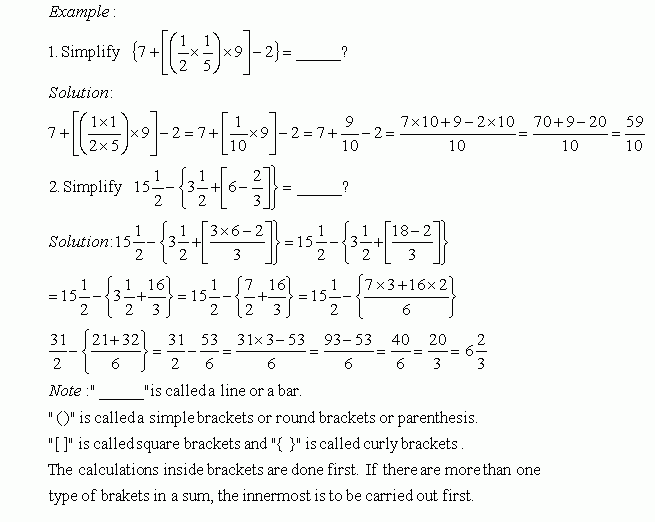Directions: Answer the following questions. Also write at least 5 examples of your own.
 Q 1: Solve the problem.1 2/32 2/32 1/3 Q 2: Solve the problem.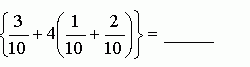1 1/21 2/32 1/4 Q 3: Solve the problem.12/911/910/9 Q 4: Solve the problem.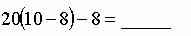313230 Q 5: Solve the problem.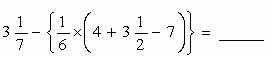4 6/603 5/842 5/80 Q 6: Solve the problem.131215 Q 7: Solve the problem.222321 Q 8: Solve the problem.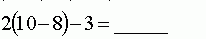201 Question 9: This question is available to subscribers only! Question 10: This question is available to subscribers only!

#### Subscription to kwizNET Learning System offers the following benefits:

• Unrestricted access to grade appropriate lessons, quizzes, & printable worksheets
• Instant scoring of online quizzes
• Progress tracking and award certificates to keep your student motivated
• Unlimited practice with auto-generated 'WIZ MATH' quizzes